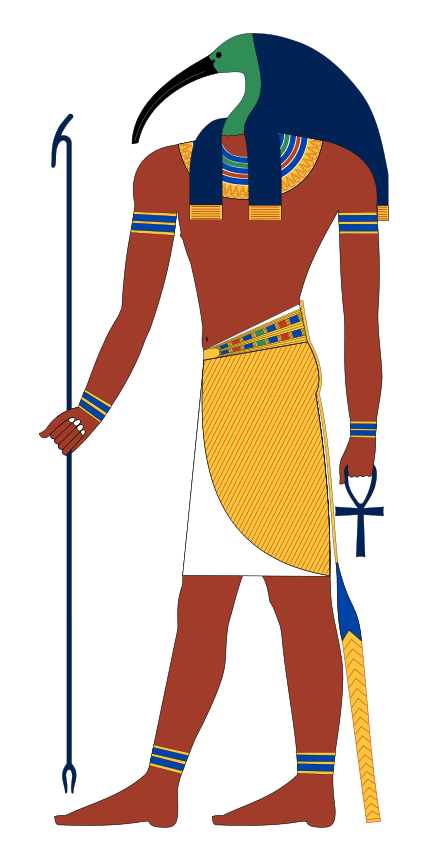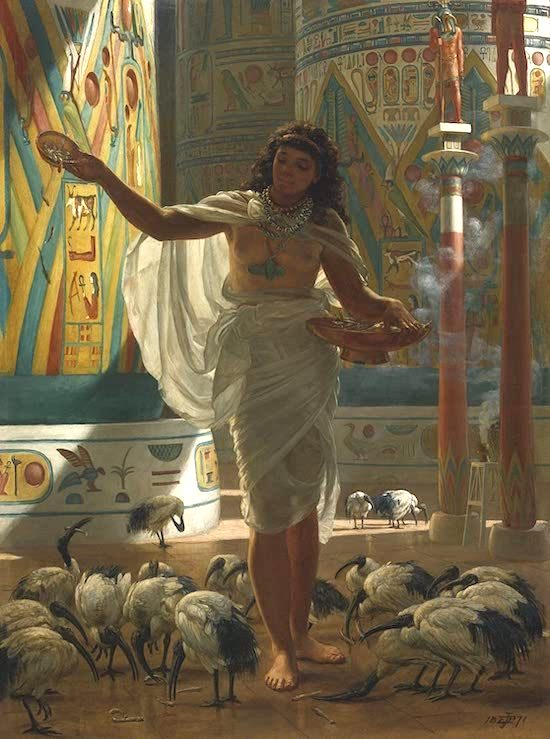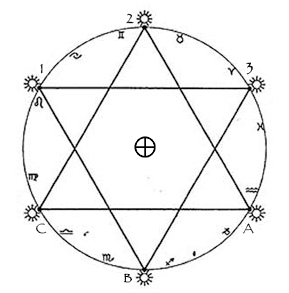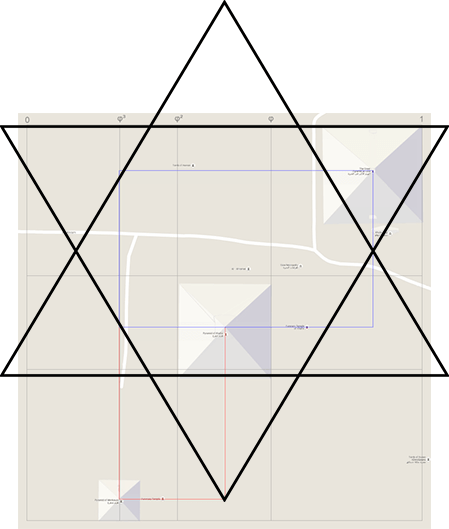# Thoth

Thoth was a master magician.Thoth is the phoenetic rendition of the sound that an ibis makes—a short, sharp, tympanic rumble that resembled the ancient Egyptian word for God. Usually described as a silent bird, the ibis makes the sound “Thoth” during its regurgitative digestion. Thoth flowed into Greek as Theos.

Thoth appears above (with the head of an ibis) and holds an Ankh and a Was Sceptre. Today we refer to the sceptre as a gnomon. Thoth was credited as being the author of every master work—human and divine. As a scribe he was overtly associated with the ibis because this bird was found along the Nile amongst papyrus plants: the wetland sedge once used to make paper.Papyrus was a woven mat of reeds that was pounded together into a hard, thin sheet. Indeed, the word paper actually comes from papyrus. The ancients noticed that when an ibis dipped its bill into the Nile it resembled a pen being dipped in ink. No surprises then, that their flight feathers were used as quill pens by the Egyptians.In the star above, we have the key conjunctions of Mercury presented as a hexagram. The superior conjunctions of 1, 2 and 3 reflect the inferior conjunctions of A, B and C. In other words, Mercury completes three orbits in under 50 weeks, so that there are six conjunctions—three superior and three inferior every year. The hexagram of Mercury therefore, was Thoth’s master symbol.

Thoth was the god of proportion, equilibrium and balance who was associated closely with the principle of Ma’at. If we examine the mythology, Thoth became Hermes who was reborn as Mercury. In light of this, we should really be measuring the solar system using the proportions of this planet:

 Planet Distancefromthe Sunkm (000) DistancewhereMercuryequals 1 Mercury 57,910 1.0000 Venus 108,200 1.8684 Earth 149,600 2.5833 Mars 227,940 3.9361 Jupiter 778,330 13.4403

Only then can the planetary relationships be seen. It’s a simple change, but this new view reveals the relationships among the planets. Each of these distance measures can be represented with an elegant pattern of simple integers from 1 to 6 appearing in roots, multipliers and exponents:

 Mercury (1) = ½ (√1+1) Mercury at aphelion = ½ ( √2 + 1 ) Venus = Mercury * (½ ( √3 + 1 )) ² Earth = Venus ¾ * (½ ( √5 + 1 )) Mars = Earth ¾ * (½ ( √6 + √2 )) Jupiter = Mars * ( √2 + 2 )

An alternate representation of the same number is used for Venus to Earth to add insight to the pattern that develops:

 Mercury=   ½(√1+1) Venus   =  (½(√3+1)) ² Earth    = ((½(√3+1))^(½(√4+1))*(½(√5+1))) Mars     = ((½(√3+1))^(½(√4+1))*(½(√5+1)))^¾*(½(√6+√2)) Jupiter =(((½(√3+1))^(½(√4+1))*(½(√5+1)))^¾*(½(√6+√2)))*(√2+2)

On average, Mercury is the closest planet to Earth—and to every other planet in the solar system. Thoth and Egyptian mythology have become the shadow of modern mathematics because we have lost the harmonics of this ancient civilisation. Indeed, our current astronomy is debauched—as proof of this just explore the astronomical unit.

Nowhere is the legacy of Thoth preserved more permanently than in the pyramids at Giza. This is hinted at by the golden mean rectangle (in blue) below that hints at the three rectangles of the internal pentagon. The pentagram therefore, remains the esoteric symbol of Thoth.The ancient Egyptians regarded Thoth as the master of spacial law, making proper use of Ma’at. He was credited with making the calculations for the establishment of the heavens and Earth and everything in between. Without his divine words, the Egyptians believed, the universe would have no meaning.